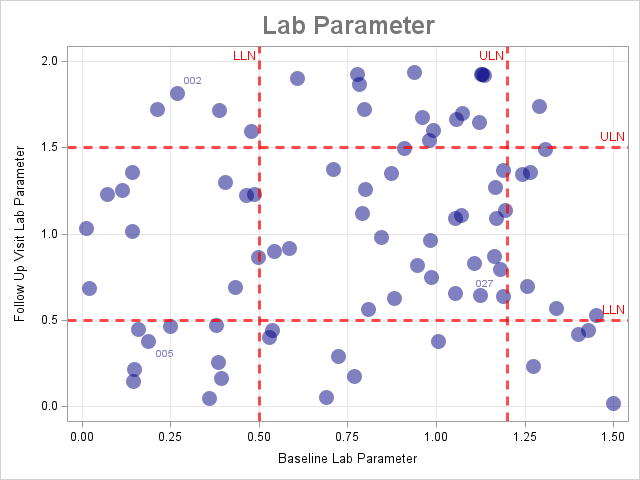## Producing shift plots with corresponding Upper Level Normal (ULN) & Lower Lever Normal (LLN)

I am new to this group.  I have instructions from a document to produce a shift plots with corresponding normal ranges.  Included are boundaries for the Upper Limit Normal (ULN) and Lower Limit Normal (LLN) [see attachment].  I tried searching the web and found very little.  This graph was produced by a document with no data to run; this is what they expect as a result of the data they will send us.  Can SAS produce this graph?  If so, how.  Any response will be greatly appreciated.

Thank you & stay safe.

1 ACCEPTED SOLUTION

Accepted Solutions

## Re: Producing shift plots with corresponding Upper Level Normal (ULN) & Lower Lever Normal (LLN)

Here's a complete example (using made-up/fake/random data), that shows more of the subtle details needed to get the exact plot you showed:

data foo;
do subject_id = 1 to 80;
x=ranuni(123)*1.5;
y=ranuni(123)*2.0;
plabel=' ';
if subject_id in (2 5 27) then plabel=trim(left(put(subject_id,z3.)));
output;
end;
run;

%let x_uln=1.2;
%let x_lln=0.5;
%let y_uln=1.5;
%let y_lln=0.5;

title1 h=18pt c=gray77 "Lab Parameter";

proc sgplot data=foo;
label y='Follow Up Visit Lab Parameter';
label x='Baseline Lab Parameter';
scatter x=x y=y / markerattrs=(size=15px color=navy symbol=circlefilled) transparency=.5
datalabel=plabel datalabelattrs=(color=navy);
refline &x_uln / axis=x lineattrs=(color=red thickness=3px pattern=dash) transparency=.3
label="ULN" labelattrs=(color=red) labelloc=inside labelpos=max;
refline &x_lln / axis=x lineattrs=(color=red thickness=3px pattern=dash) transparency=.3
label="LLN" labelattrs=(color=red) labelloc=inside labelpos=max;
refline &y_uln / axis=y lineattrs=(color=red thickness=3px pattern=dash) transparency=.3
label="ULN" labelattrs=(color=red) labelloc=inside labelpos=max;
refline &y_lln / axis=y lineattrs=(color=red thickness=3px pattern=dash) transparency=.3
label="LLN" labelattrs=(color=red) labelloc=inside labelpos=max;
xaxis grid;
yaxis grid;
run;2 REPLIES 2

## Re: Producing shift plots with corresponding Upper Level Normal (ULN) & Lower Lever Normal (LLN)

It looks like the plot plots the clinical “normal ranges” of the lab values. These

values appear to be approximately 0.55 and 1.5 for this value. Check the source of this plot to find out the exact values you should use.

So you can create a plot like this by using PROC SGPLOT to create a scatter plot and then using the REFLINE statement to add the reference lines on each axis:

proc sgplot data=Have;

scatter x=x y=y;

refline 0.55 1.5 / axis=x;  /* normal clinical range */

refline 0.55 1.5 / axis=y;  /* percentiles from PROC MEANS */

xaxis min=0 grid;

yaxis min=0 grid;

run;

## Re: Producing shift plots with corresponding Upper Level Normal (ULN) & Lower Lever Normal (LLN)

Here's a complete example (using made-up/fake/random data), that shows more of the subtle details needed to get the exact plot you showed:

data foo;
do subject_id = 1 to 80;
x=ranuni(123)*1.5;
y=ranuni(123)*2.0;
plabel=' ';
if subject_id in (2 5 27) then plabel=trim(left(put(subject_id,z3.)));
output;
end;
run;

%let x_uln=1.2;
%let x_lln=0.5;
%let y_uln=1.5;
%let y_lln=0.5;

title1 h=18pt c=gray77 "Lab Parameter";

proc sgplot data=foo;
label y='Follow Up Visit Lab Parameter';
label x='Baseline Lab Parameter';
scatter x=x y=y / markerattrs=(size=15px color=navy symbol=circlefilled) transparency=.5
datalabel=plabel datalabelattrs=(color=navy);
refline &x_uln / axis=x lineattrs=(color=red thickness=3px pattern=dash) transparency=.3
label="ULN" labelattrs=(color=red) labelloc=inside labelpos=max;
refline &x_lln / axis=x lineattrs=(color=red thickness=3px pattern=dash) transparency=.3
label="LLN" labelattrs=(color=red) labelloc=inside labelpos=max;
refline &y_uln / axis=y lineattrs=(color=red thickness=3px pattern=dash) transparency=.3
label="ULN" labelattrs=(color=red) labelloc=inside labelpos=max;
refline &y_lln / axis=y lineattrs=(color=red thickness=3px pattern=dash) transparency=.3
label="LLN" labelattrs=(color=red) labelloc=inside labelpos=max;
xaxis grid;
yaxis grid;
run;Discussion stats
• 2 replies
• 835 views
• 2 likes
• 3 in conversation